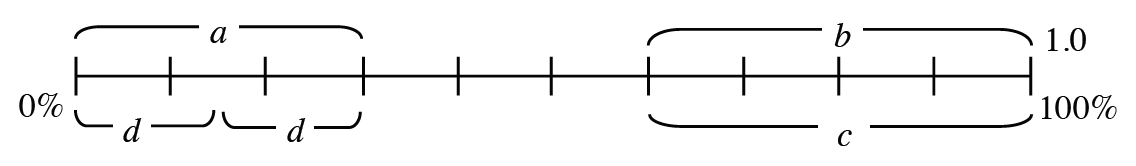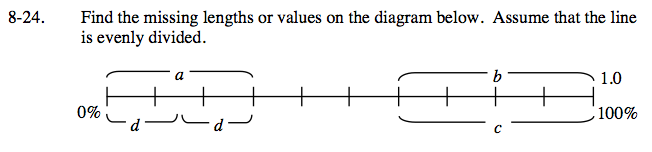### Home > CC2 > Chapter 8 > Lesson 8.1.2 > Problem8-24

8-24.

Find the missing lengths or values on the diagram below. Assume that the line is evenly divided. Homework Help ✎How many sections is this line divided into? Can you use this to find the value of each section?

Since the line is divided into 10 sections, the sections each represent 10% or 0.1 of the whole.

To solve, note how many sections each bracket includes.

a. 0.3
b. Use the method above to solve.
c. 40%
d. Use the method above to solve.
Note that the two sections are equal.